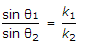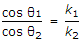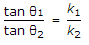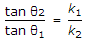# Civil Engineering - Soil Mechanics and Foundation Engineering

Exercise : Soil Mechanics and Foundation Engineering - Section 1
11.
Minimum size of the particles of silt soil, is
0.002 mm
0.04 mm
0.06 mm.
0.08 mm
1 mm
Explanation:
No answer description is available. Let's discuss.

12.
The maximum value of effective stress in the past divided by the present value, is defined as over consolidation ratio (OCR). The O.C.R. of an over consolidated clay is
less than 1
1
more than 1
None of these.
Explanation:
No answer description is available. Let's discuss.

13.
If Nf, Nd and H are total number flow channels, total number of potential drops and total hydraulic head differences respectively, the discharge q through the complete flow is given by (where K is a constant)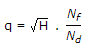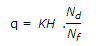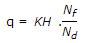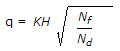Explanation:
No answer description is available. Let's discuss.

14.
A flow line makes angles θ1 and θ2 with the normal to the interface of the soils having permeabilities k1, k2 before and after deflection. According to the law of deflection of the flow lines at the interface of the dissimilar soils